### 手机扫一扫访问本页内容

##### 微信扫一扫可打开小程序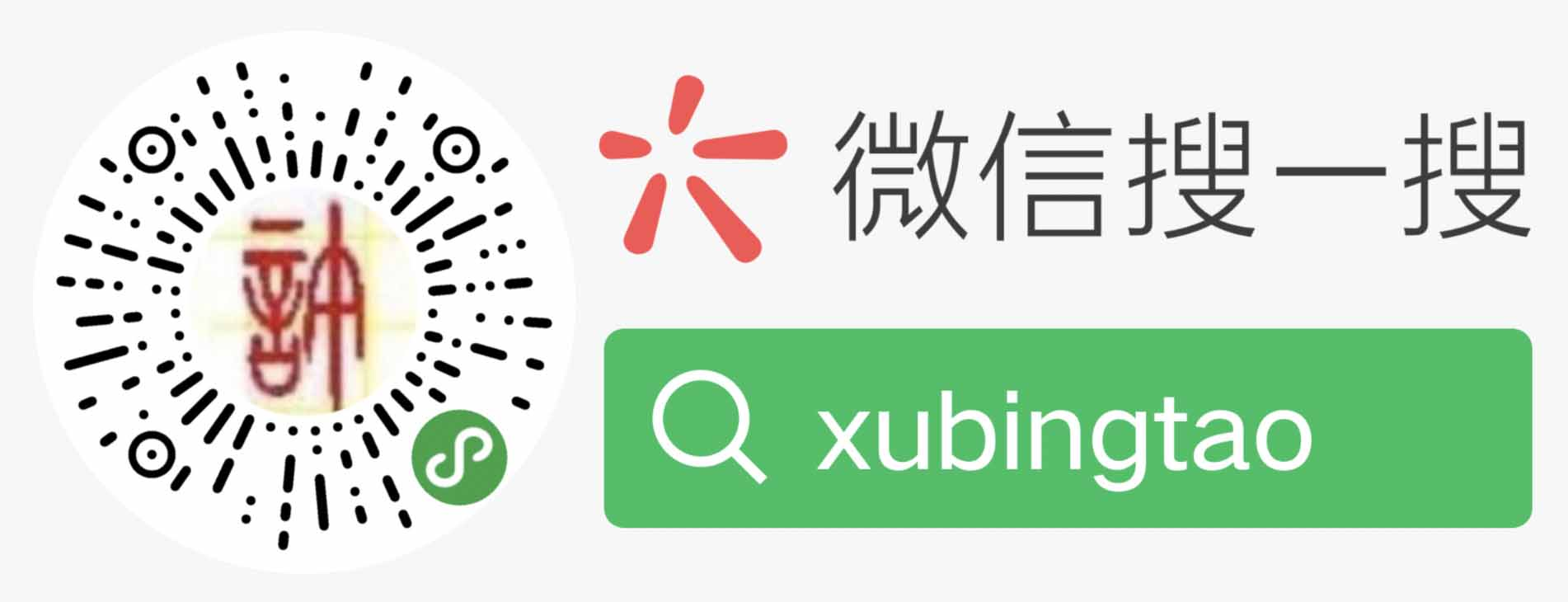## 【面试题】互换两个变量的值

``````int a=1;
int b=2;``````

``````a = a + b;
b = a - b;
a = a - b;``````

``b = (a + b) - (a = b);``

``````a = a ^ b;
b = a ^ b;//a ^ b ^ b = a
a = a ^ b;//a ^ b ^ a = b``````

``````int c = a;
a = b;
b = c;``````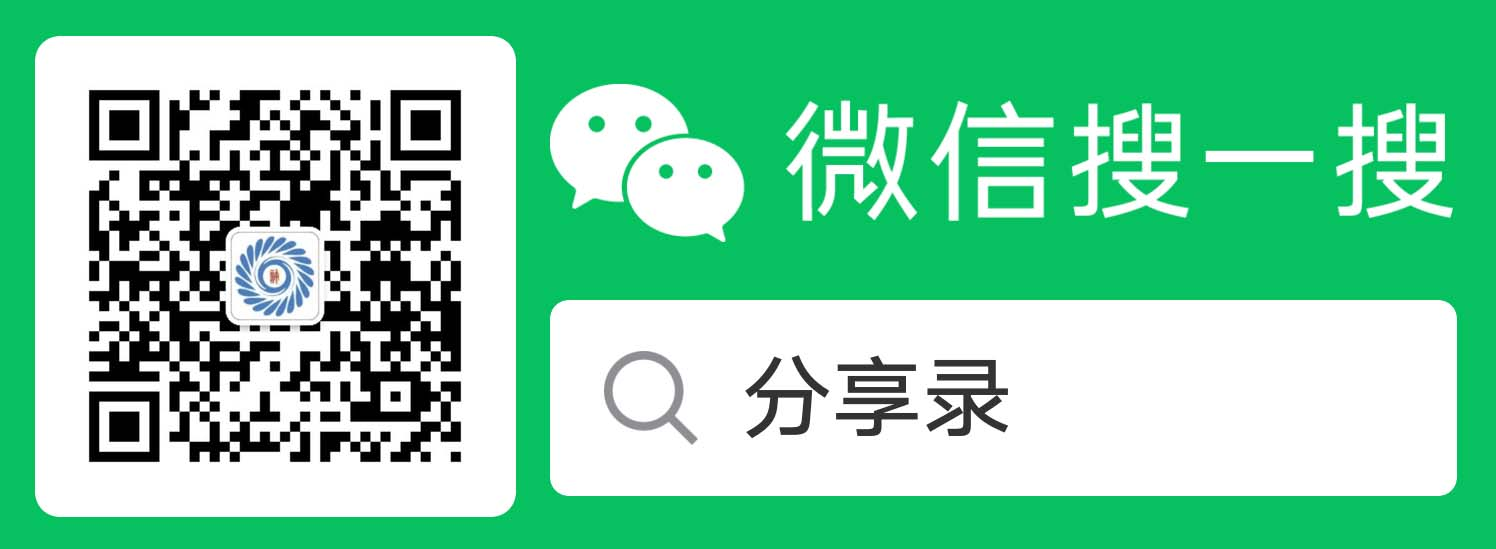关注我的公众号每天为您分享各类有用信息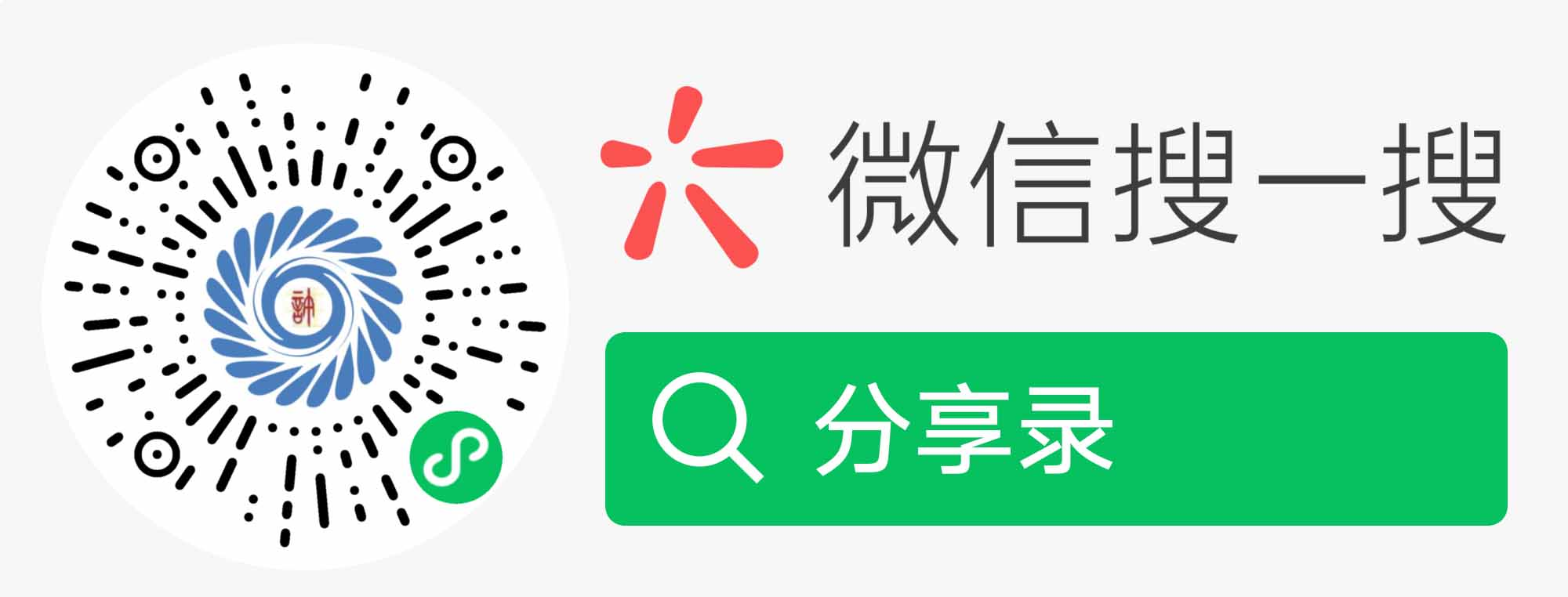扫码打开小程序可评论文章保存图片，在“我的”有实时在线客服哦，看效果？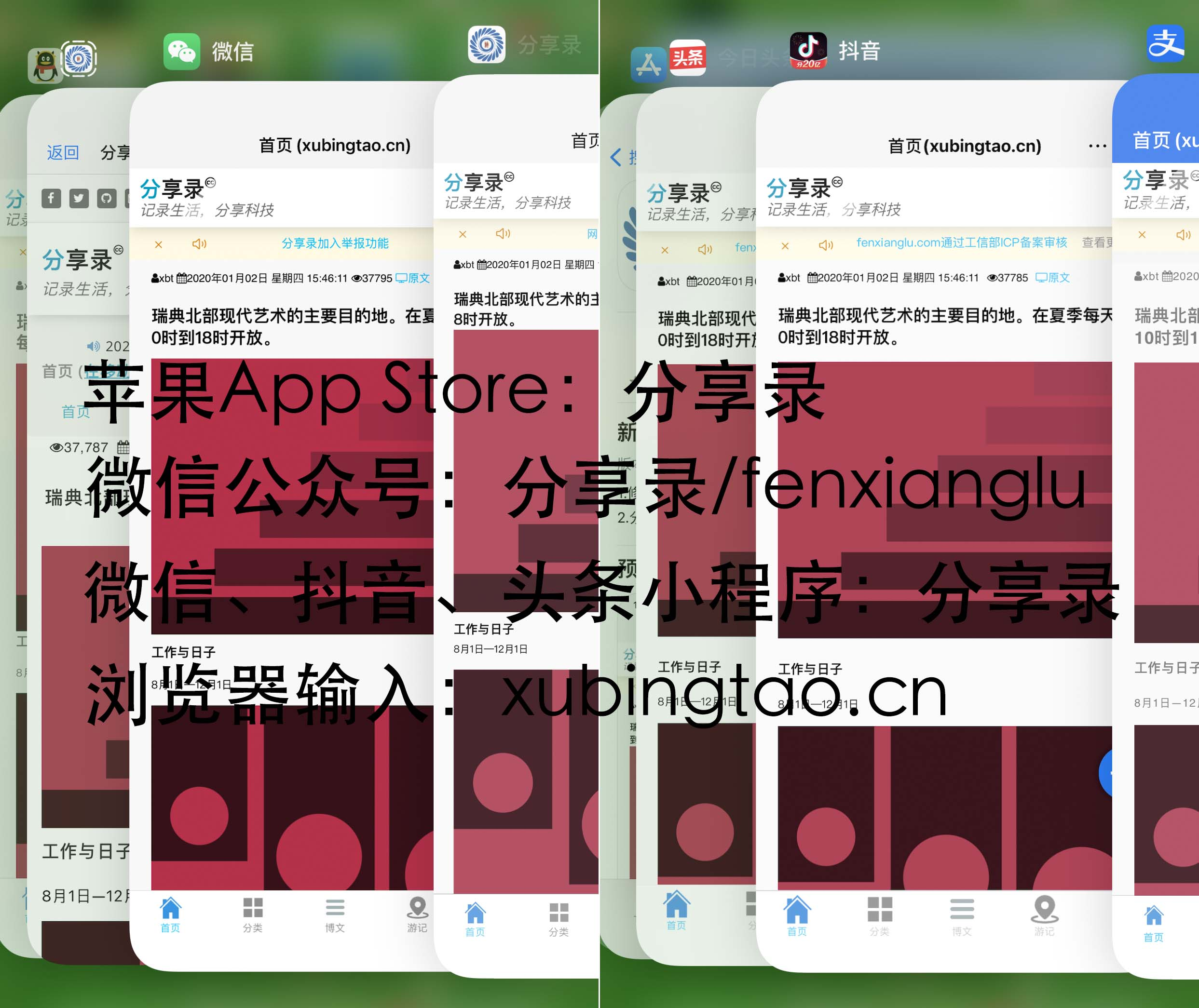分享录多端跨平台系统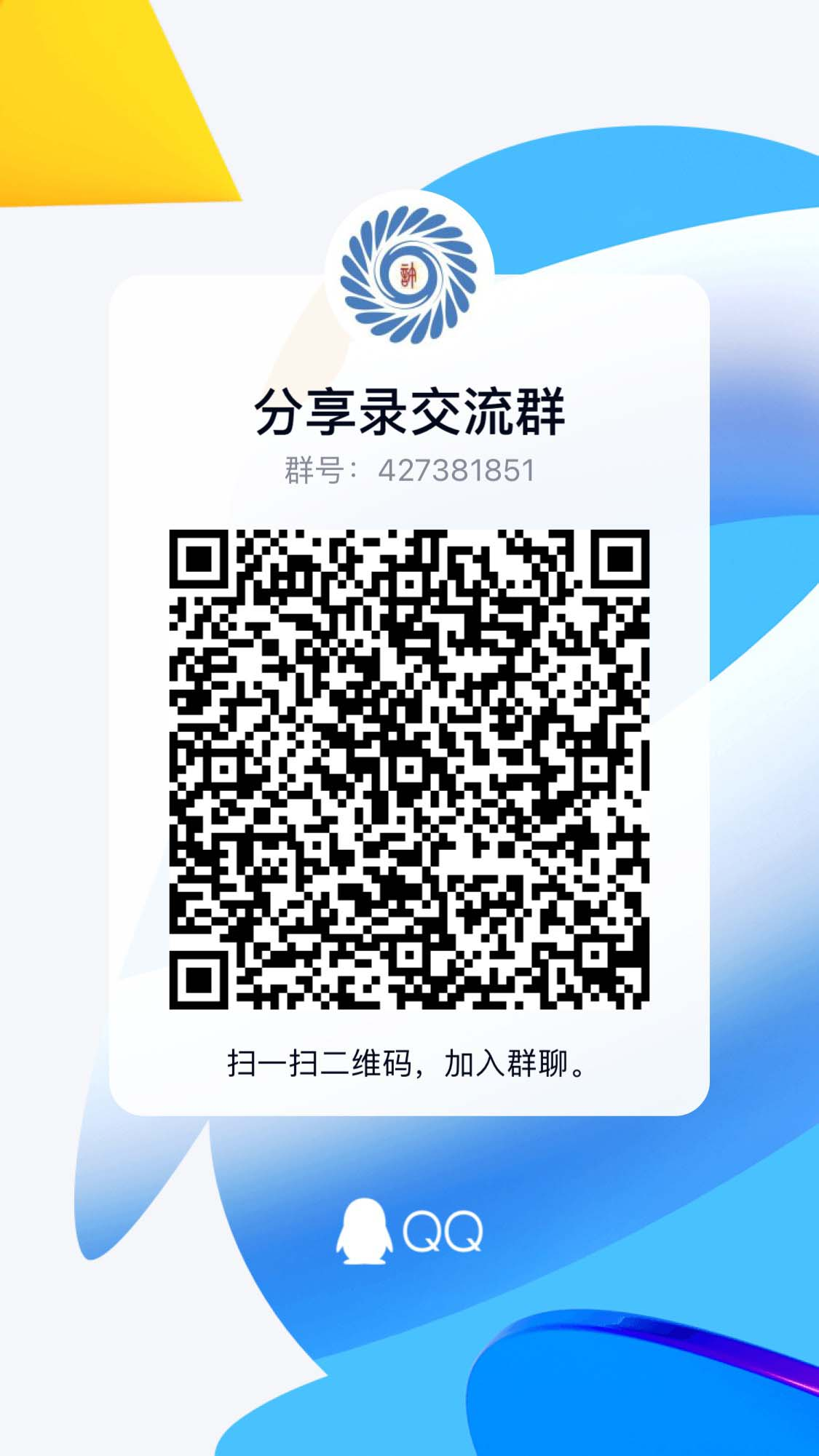分享录交流群